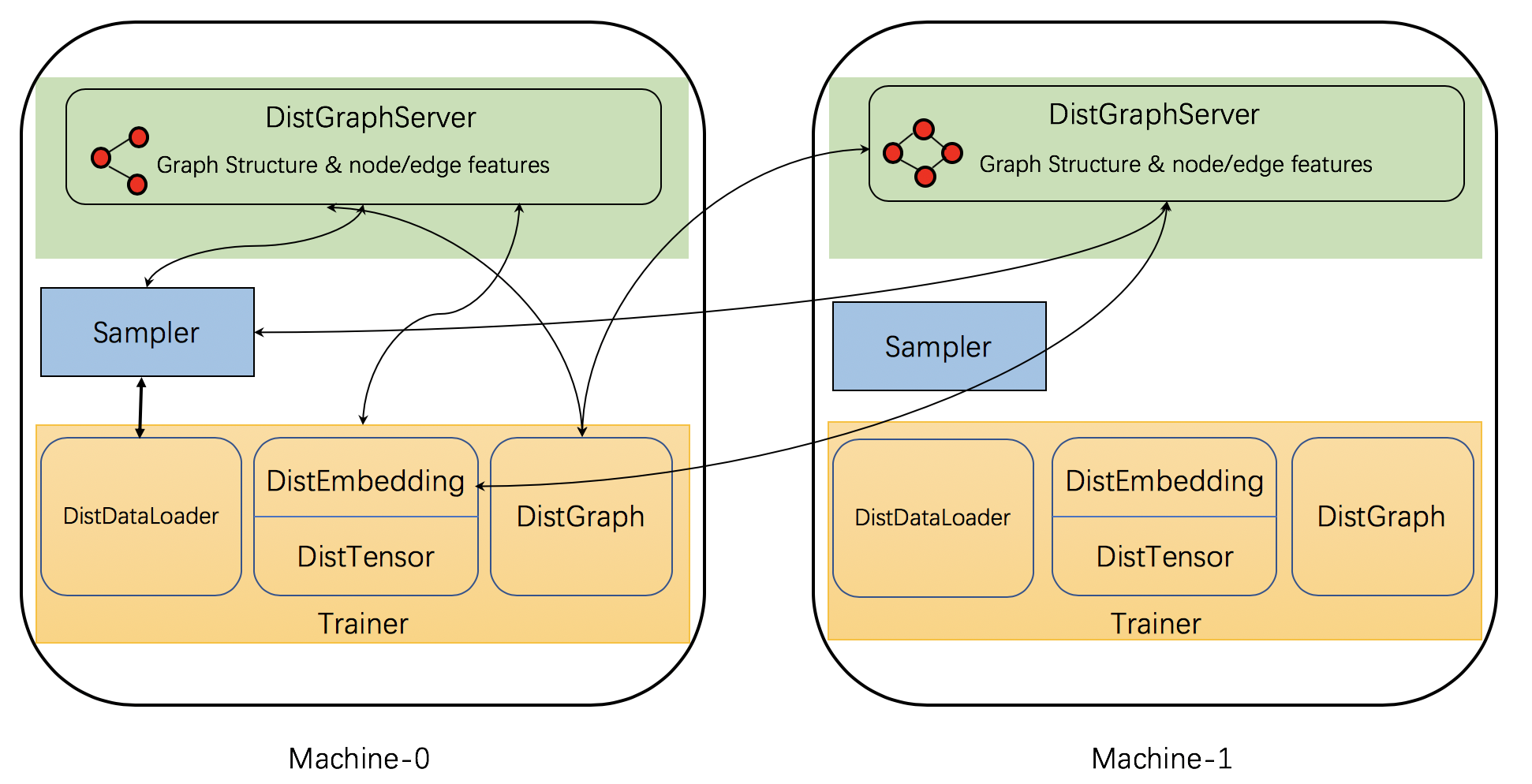# Chapter 7: Distributed Training¶

(中文版)

DGL adopts a fully distributed approach that distributes both data and computation across a collection of computation resources. In the context of this section, we will assume a cluster setting (i.e., a group of machines). DGL partitions a graph into subgraphs and each machine in a cluster is responsible for one subgraph (partition). DGL runs an identical training script on all machines in the cluster to parallelize the computation and runs servers on the same machines to serve partitioned data to the trainers.

For the training script, DGL provides distributed APIs that are similar to the ones for mini-batch training. This makes distributed training require only small code modifications from mini-batch training on a single machine. Below shows an example of training GraphSage in a distributed fashion. The only code modifications are located on line 4-7: 1) initialize DGL’s distributed module, 2) create a distributed graph object, and 3) split the training set and calculate the nodes for the local process. The rest of the code, including sampler creation, model definition, training loops are the same as mini-batch training.

import dgl
import torch as th

dgl.distributed.initialize('ip_config.txt')
th.distributed.init_process_group(backend='gloo')
g = dgl.distributed.DistGraph('graph_name', 'part_config.json')
pb = g.get_partition_book()
train_nid = dgl.distributed.node_split(g.ndata['train_mask'], pb, force_even=True)

# Create sampler
sampler = NeighborSampler(g, [10,25],
dgl.distributed.sample_neighbors,
device)

dataset=train_nid.numpy(),
batch_size=batch_size,
collate_fn=sampler.sample_blocks,
shuffle=True,
drop_last=False)

# Define model and optimizer
model = SAGE(in_feats, num_hidden, n_classes, num_layers, F.relu, dropout)
model = th.nn.parallel.DistributedDataParallel(model)
loss_fcn = nn.CrossEntropyLoss()
optimizer = optim.Adam(model.parameters(), lr=args.lr)

# training loop
for epoch in range(args.num_epochs):
for step, blocks in enumerate(dataloader):
batch_inputs, batch_labels = load_subtensor(g, blocks.srcdata[dgl.NID],
blocks[-1].dstdata[dgl.NID])
batch_pred = model(blocks, batch_inputs)
loss = loss_fcn(batch_pred, batch_labels)
loss.backward()
optimizer.step()


When running the training script in a cluster of machines, DGL provides tools to copy data to the cluster’s machines and launch the training job on all machines.

Note: The current distributed training API only supports the Pytorch backend.

DGL implements a few distributed components to support distributed training. The figure below shows the components and their interactions.Specifically, DGL’s distributed training has three types of interacting processes: server, sampler and trainer.

• Server processes run on each machine that stores a graph partition (this includes the graph structure and node/edge features). These servers work together to serve the graph data to trainers. Note that one machine may run multiple server processes simultaneously to parallelize computation as well as network communication.

• Sampler processes interact with the servers and sample nodes and edges to generate mini-batches for training.

• Trainers contain multiple classes to interact with servers. It has DistGraph to get access to partitioned graph data and has DistEmbedding and DistTensor to access the node/edge features/embeddings. It has DistDataLoader to interact with samplers to get mini-batches.

Having the distributed components in mind, the rest of the section will cover the following distributed components: